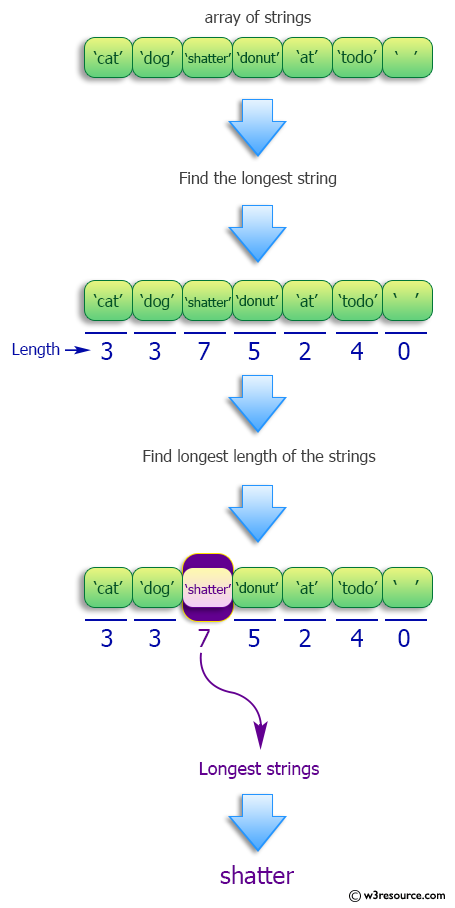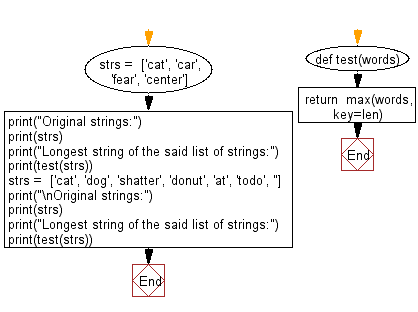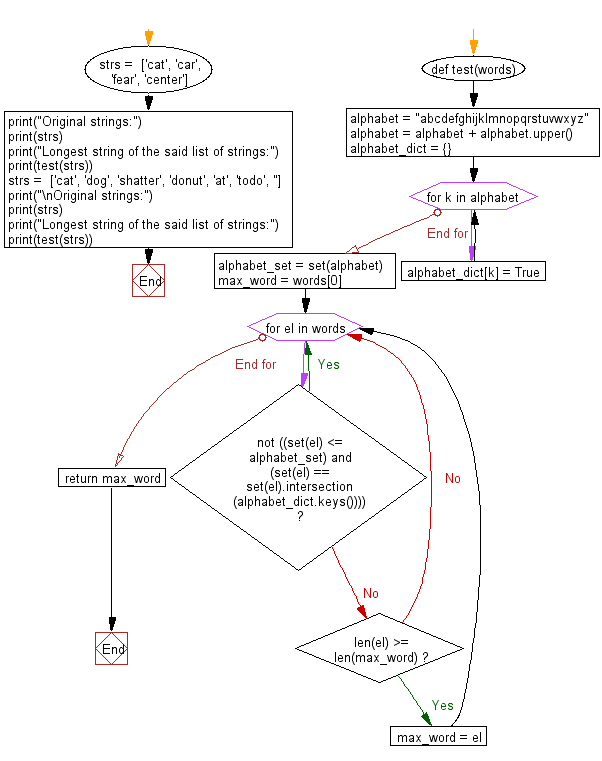﻿ Python: Find the longest string of a list of strings - w3resource# Python: Find the longest string of a list of strings

## Python Programming Puzzles: Exercise-15 with Solution

Write a Python program find the longest string of a given list of strings.

```Input:
['cat', 'car', 'fear', 'center']
Output:
center

Input:
['cat', 'dog', 'shatter', 'donut', 'at', 'todo', '']
Output:
shatter
```

Pictorial Presentation:Sample Solution-1:

Python Code:

``````def test(words):
return  max(words, key=len)
strs =  ['cat', 'car', 'fear', 'center']
print("Original strings:")
print(strs)
print("Longest string of the said list of strings:")
print(test(strs))
strs =  ['cat', 'dog', 'shatter', 'donut', 'at', 'todo', '']
print("\nOriginal strings:")
print(strs)
print("Longest string of the said list of strings:")
print(test(strs))
``````

Sample Output:

```Original strings:
['cat', 'car', 'fear', 'center']
Longest string of the said list of strings:
center

Original strings:
['cat', 'dog', 'shatter', 'donut', 'at', 'todo', '']
Longest string of the said list of strings:
shatter
```

Flowchart:## Visualize Python code execution:

The following tool visualize what the computer is doing step-by-step as it executes the said program:

Sample Solution-2:

Python Code:

``````#License: https://bit.ly/3oLErEI

def test(words):
alphabet = "abcdefghijklmnopqrstuvwxyz"
alphabet = alphabet + alphabet.upper()
alphabet_dict = {}
for k in alphabet:
alphabet_dict[k] = True
alphabet_set = set(alphabet)
max_word = words
for el in words:
if not ((set(el) <= alphabet_set) and (set(el) == set(el).intersection(alphabet_dict.keys()))):
continue
if len(el) >= len(max_word):
max_word = el
return max_word
strs =  ['cat', 'car', 'fear', 'center']
print("Original strings:")
print(strs)
print("Longest string of the said list of strings:")
print(test(strs))
strs =  ['cat', 'dog', 'shatter', 'donut', 'at', 'todo', '']
print("\nOriginal strings:")
print(strs)
print("Longest string of the said list of strings:")
print(test(strs))
``````

Sample Output:

```Original strings:
['cat', 'car', 'fear', 'center']
Longest string of the said list of strings:
center

Original strings:
['cat', 'dog', 'shatter', 'donut', 'at', 'todo', '']
Longest string of the said list of strings:
shatter
```

Flowchart:## Visualize Python code execution:

The following tool visualize what the computer is doing step-by-step as it executes the said program:

Python Code Editor :

Have another way to solve this solution? Contribute your code (and comments) through Disqus.

What is the difficulty level of this exercise?

Test your Programming skills with w3resource's quiz.

﻿

## Python: Tips of the Day

Clamps num within the inclusive range specified by the boundary values x and y:

Example:

```def tips_clamp_num(num,x,y):
return max(min(num, max(x, y)), min(x, y))
print(tips_clamp_num(2, 4, 6))
print(tips_clamp_num(1, -1, -6))
```

Output:

```4
-1
```